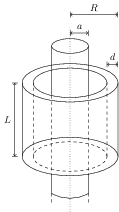# Faraday's Law

## Problems from IIT JEE

Problem (IIT JEE 2005):A long solenoid of radius $a$ and number of turns per unit length $n$ is enclosed by cylindrical shell of radius $R$, thickness $d\ (d\ll R)$ and length $L$. A variable current $i=i_0 \sin\omega t$ flows through the solenoid. If the resistivity of the material of cylindrical shell is $\rho$, find the induced current in the shell.

Solution: The magnetic field inside the solenoid is $B=\mu_0 n i$ (along its axis) and outside the solenoid it is zero. Thus, flux passing through the cross-section of cylinder is, \begin{alignat}{2} &\phi=\int \vec{B}\cdot\mathrm{d}\vec{S}=\mu_0 n i_0\sin\omega t (\pi a^2). \nonumber \end{alignat} By Faraday's law, the induced emf is given by, \begin{alignat}{2} &e=-\mathrm{d}\phi/\mathrm{d}t=-\pi a^2 \mu_0 n i_0 \omega \cos\omega t. \nonumber \end{alignat} The emf $e$ induces circumferential current in the cylinder. The cylinder resistance for circumferential currents is $\rho l/A$ where $l=2\pi R$ and $A=Ld$. The induced current is given by, \begin{alignat}{2} i=\frac{e}{\rho l/A}=\frac{\mu_0 L d n a^2 i_0 \omega \cos\omega t}{2\rho R}. \nonumber \end{alignat}